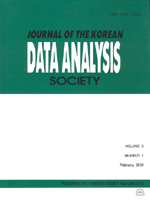상세검색
최근 검색어 전체 삭제
다국어입력
즐겨찾기0KCI등재 학술저널

# Alternative Expressions of Regression Vector for Principal Component Regression and Partial Least Squares Regression

• 등재여부 : KCI등재
• 2003.03
• 17 - 26 (10 pages)

Principal component regression (PCR) and partial least squares regression (PLSR) are examined. They usually appear in completely different computational forms in the literature. Since PLSR procedure is always given as an iterative algorithm, it is difficult to see its relationship with PCR. In this paper, alternative unifying expressions of the regression vectors for PCR and PLSR are derived using singular value decomposition of the predictor variables matrix. They are non-iterative and comparative expressions and enable us to gain some insight into the interrelationships between those methods.

1. Introduction

2. Development of Alternative Expressions

3. Comparative Expressions

4. Summary

References